This is “Quadratic Formula”, section 9.3 from the book Beginning Algebra (v. 1.0). For details on it (including licensing), click here.

Has this book helped you? Consider passing it on:
Creative Commons supports free culture from music to education. Their licenses helped make this book available to you.
DonorsChoose.org helps people like you help teachers fund their classroom projects, from art supplies to books to calculators.

### Learning Objective

In this section, we will develop a formula that gives the solutions to any quadratic equation in standard form. To do this, we begin with a general quadratic equation in standard form and solve for x by completing the square. Here a, b, and c are real numbers and $a≠0$: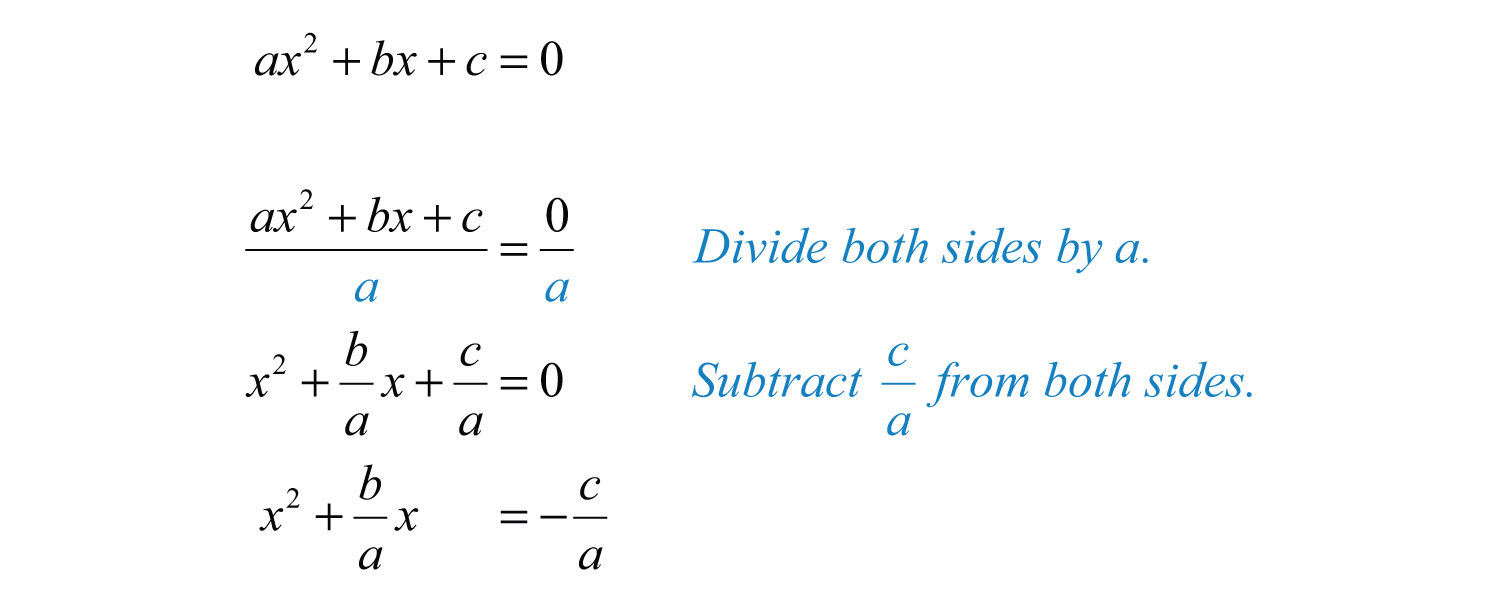Determine the constant that completes the square: take the coefficient of x, divide it by 2, and then square it.Add this to both sides of the equation and factor.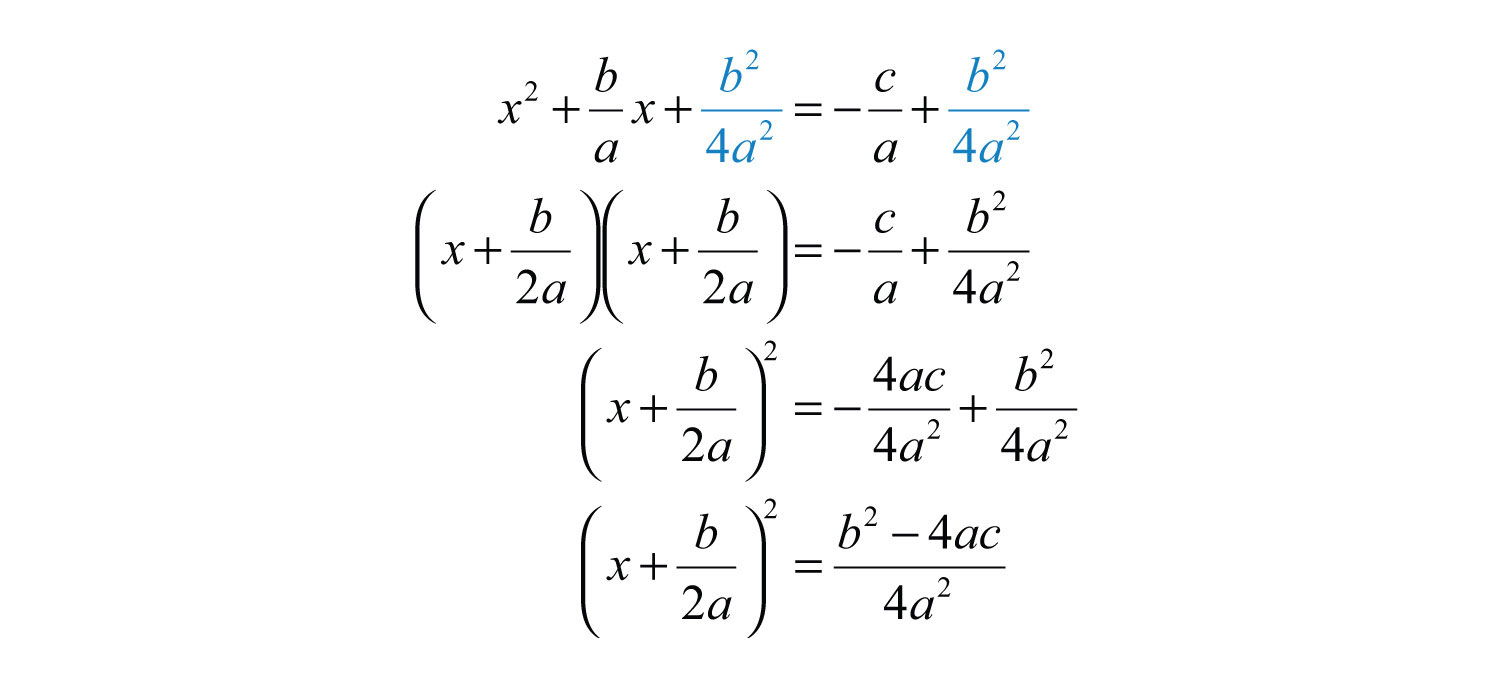Solve by extracting roots.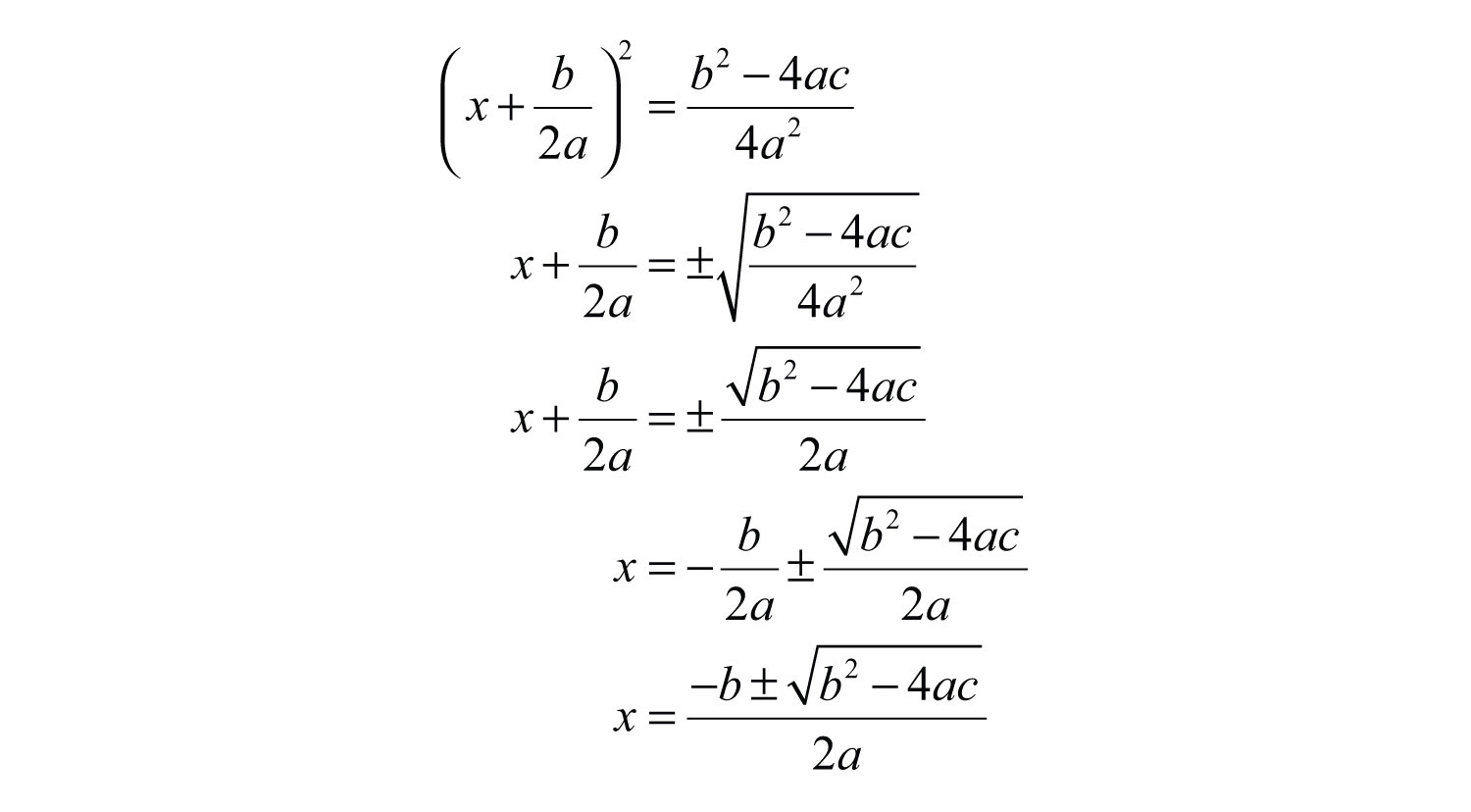This derivation gives us a formula that solves any quadratic equation in standard form. Given $ax2+bx+c=0$, where a, b, and c are real numbers and $a≠0$, then the solutions can be calculated using the quadratic formulaThe formula $x=−b±b2−4ac2a$, which gives the solutions to any quadratic equation in the form $ax2+bx+c=0$, where a, b, and c are real numbers and $a≠0$.:Consider the quadratic equation $2x2−7x+3=0$. It can be solved by factoring as follows: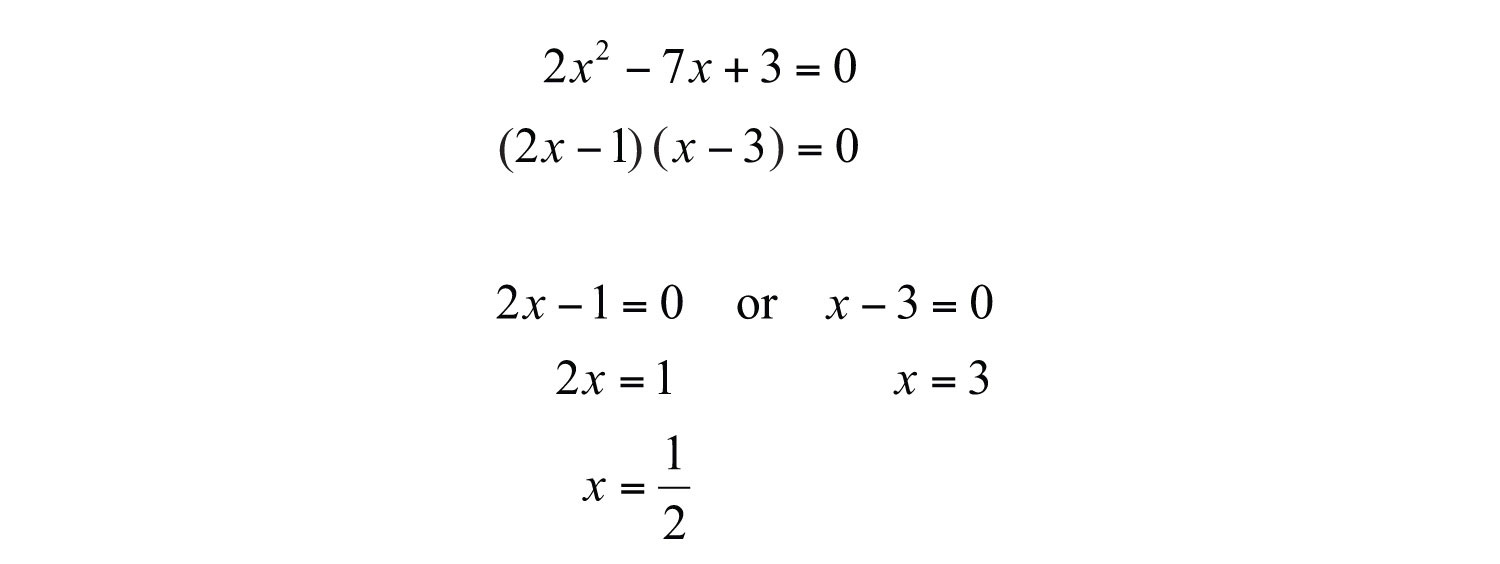The solutions are 1/2 and 3. The following example shows that we can obtain the same results using the quadratic formula.

Example 1: Solve using the quadratic formula: $2x2−7x+3=0$.

Solution: Begin by identifying a, b, and c as the coefficients of each term.Substitute these values into the quadratic formula and then simplify.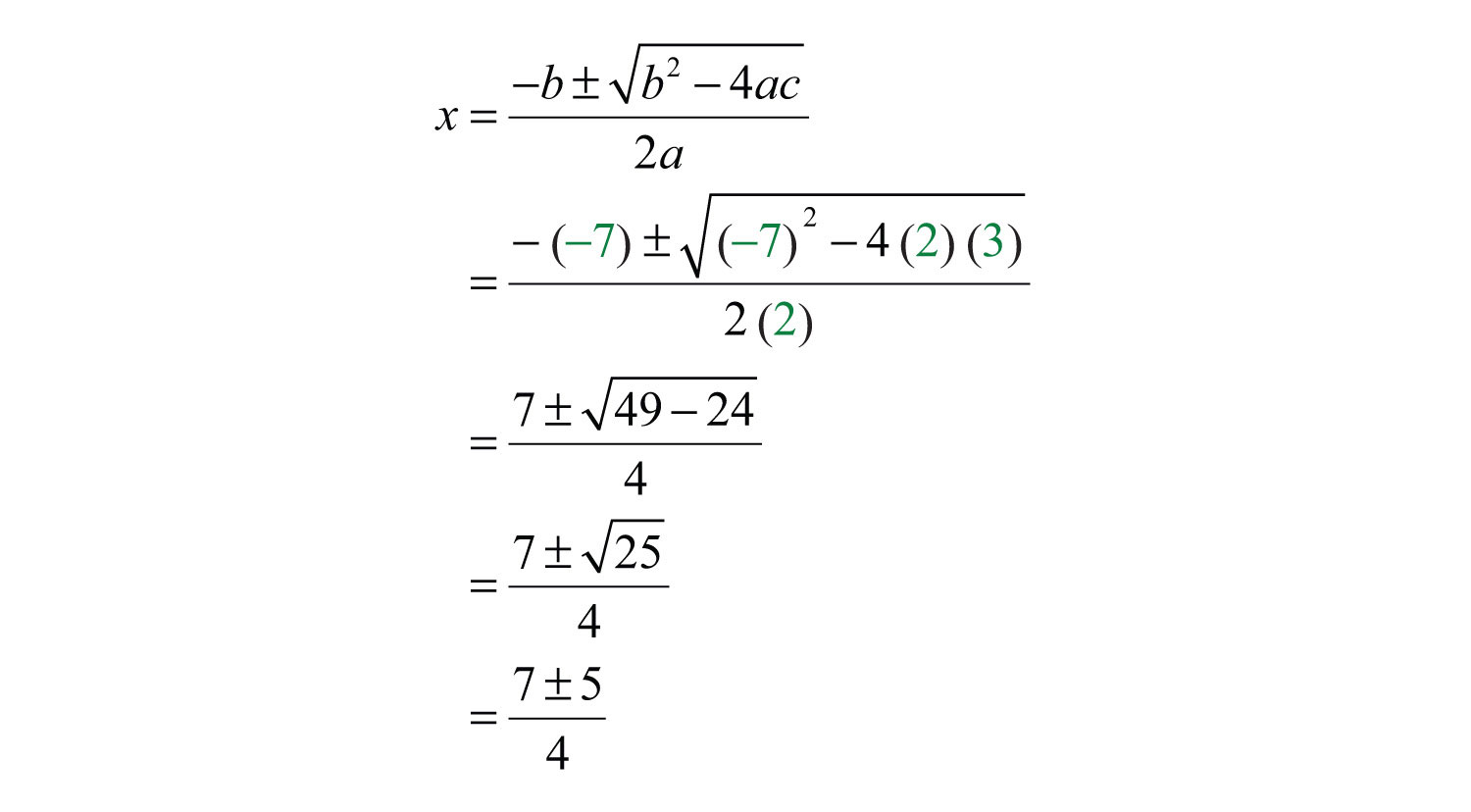Separate the “plus or minus” into two equations and simplify each individually.Answer: The solutions are 1/2 and 3.

Of course, if the quadratic factors, then it is a best practice to solve it by factoring. However, not all quadratic polynomials factor; nevertheless, the quadratic formula provides us with a means to solve such equations.

Example 2: Solve using the quadratic formula: $5x2+2x−1=0$.

Solution: Begin by identifying a, b, and c.Substitute these values into the quadratic formula.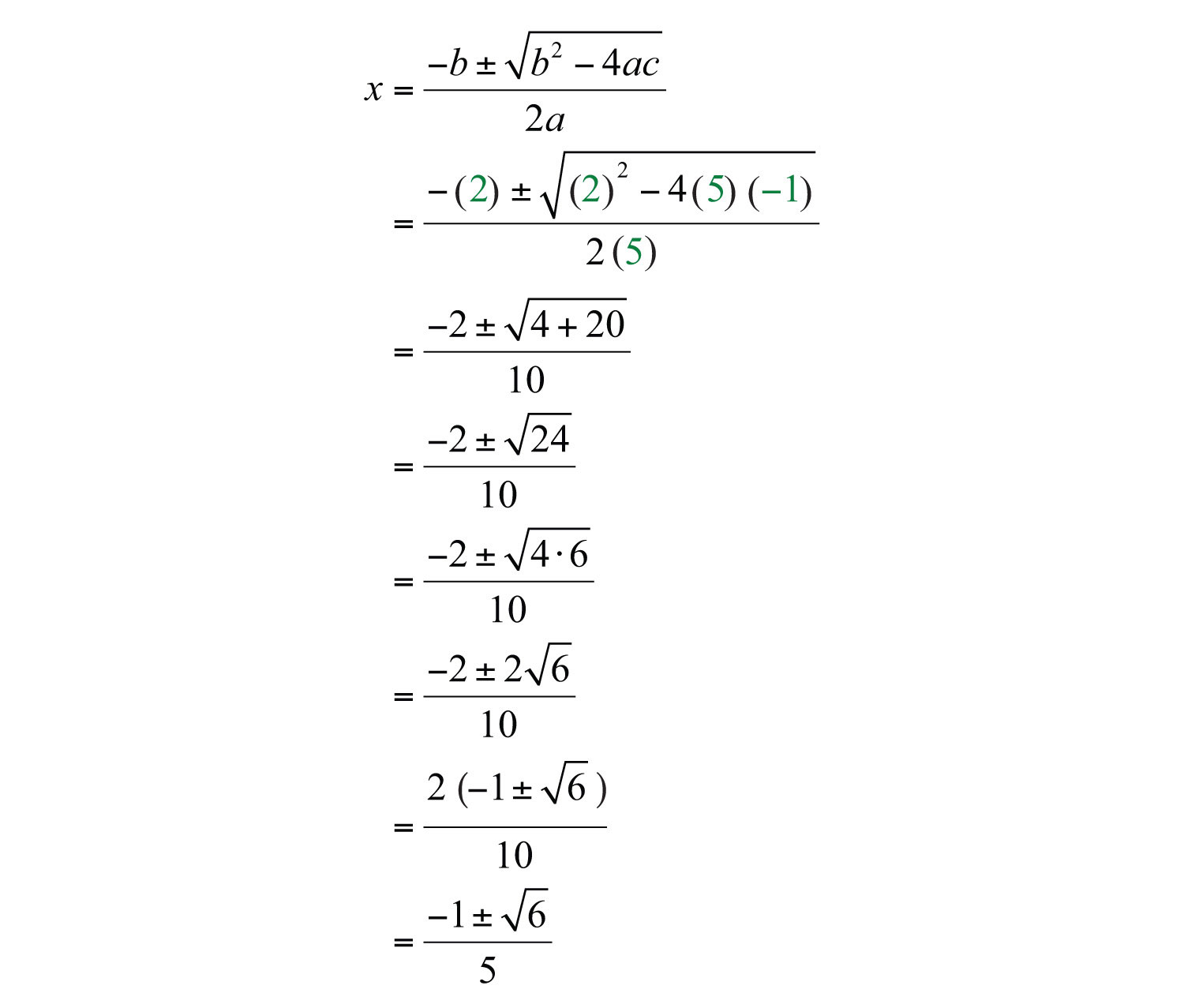Answer: The solutions are $−1±65$.

Often terms are missing. When this is the case, use 0 as the coefficient.

Example 3: Solve using the quadratic formula: $x2−18=0$.

Solution: Think of this equation with the following coefficients:HereSubstitute these values into the quadratic formula.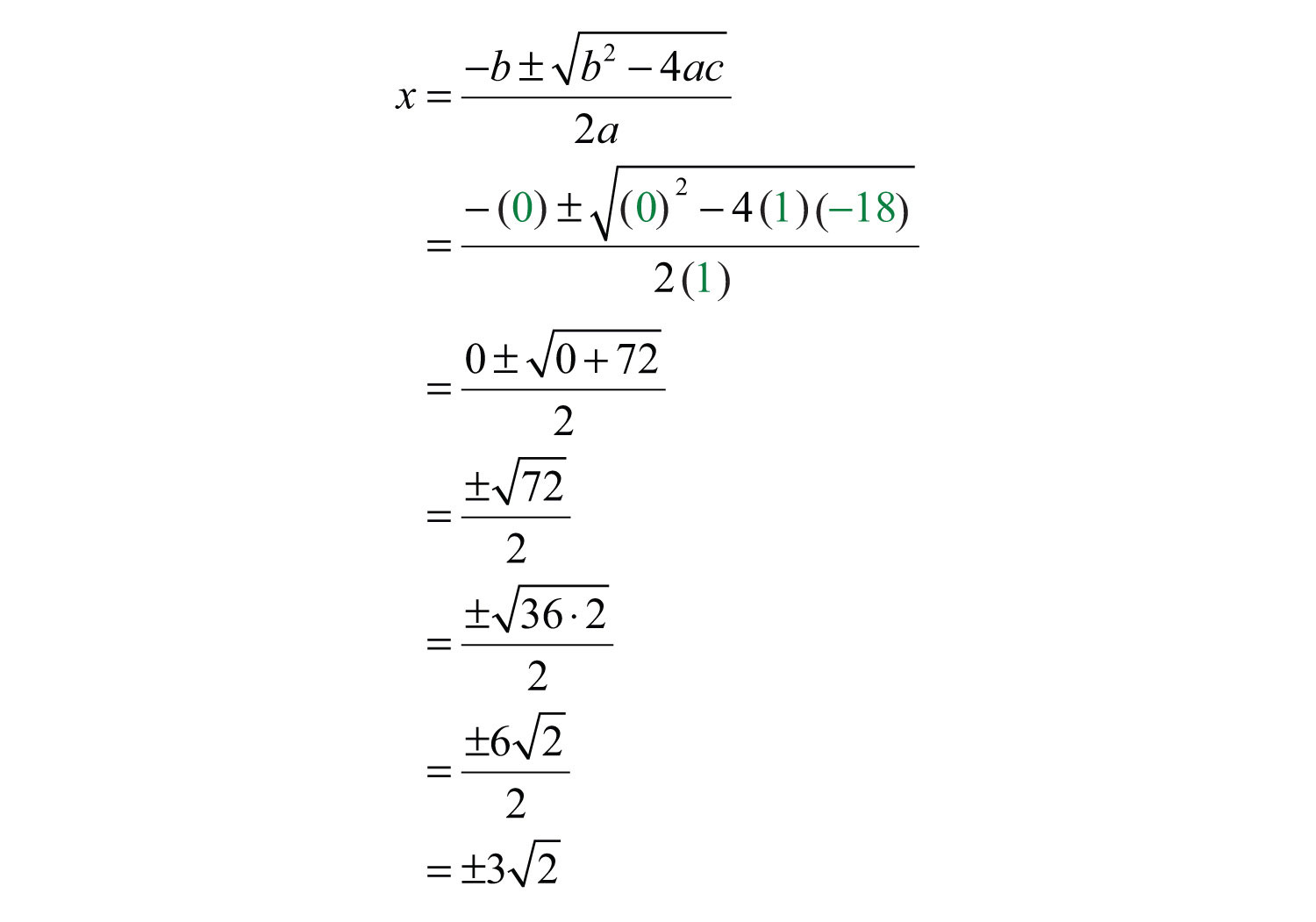Answer: The solutions are $±32$.

Since the coefficient of x was 0, we could have solved the equation by extracting the roots. As an exercise, solve the previous example using this method and verify that the results are the same.

Example 4: Solve using the quadratic formula: $9x2−12x+4=0$.

Solution: In this case,Substitute these values into the quadratic formula and then simplify.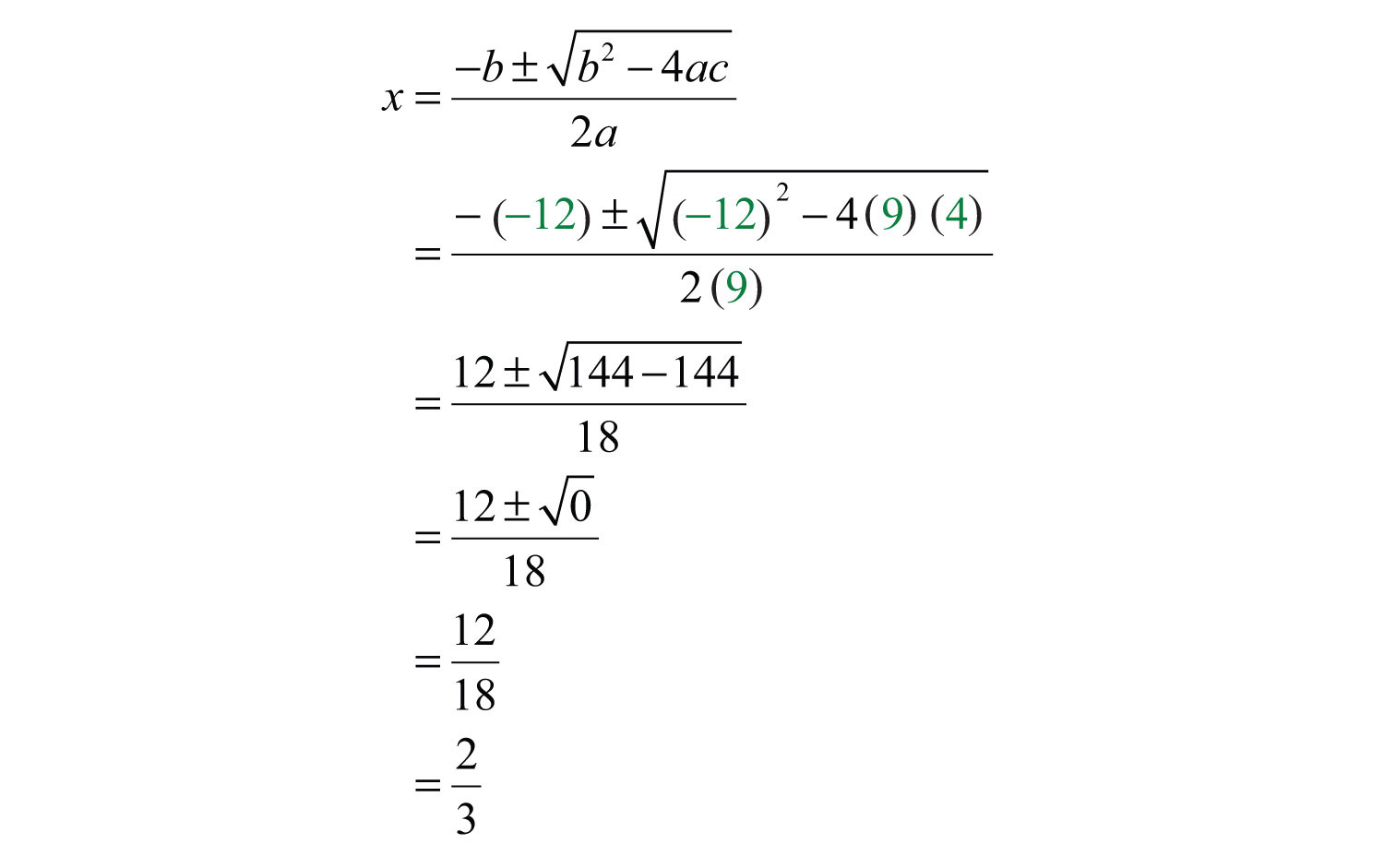In this example, notice that the radicand of the square root is 0. This results in only one solution to this quadratic equation. Normally, we expect two solutions. When we find only one solution, the solution is called a double root. If we solve this equation by factoring, then the solution appears twice.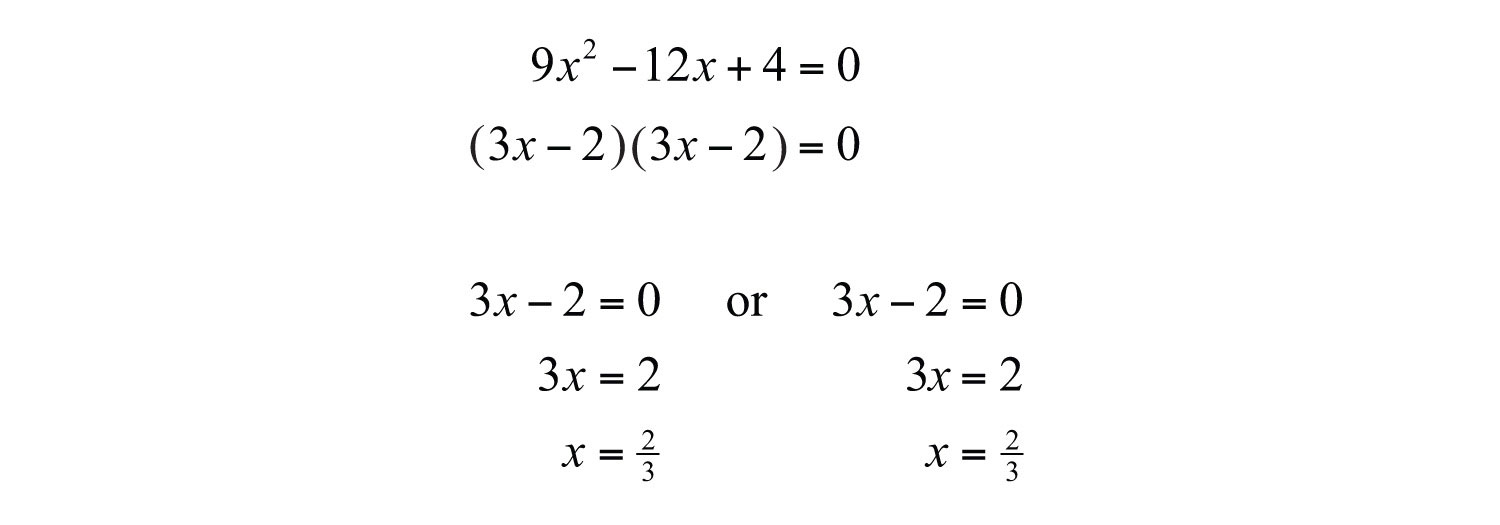Example 5: Solve using the quadratic formula: $x2+x+1=0$.

Solution: In this case,Substitute these values into the quadratic formula.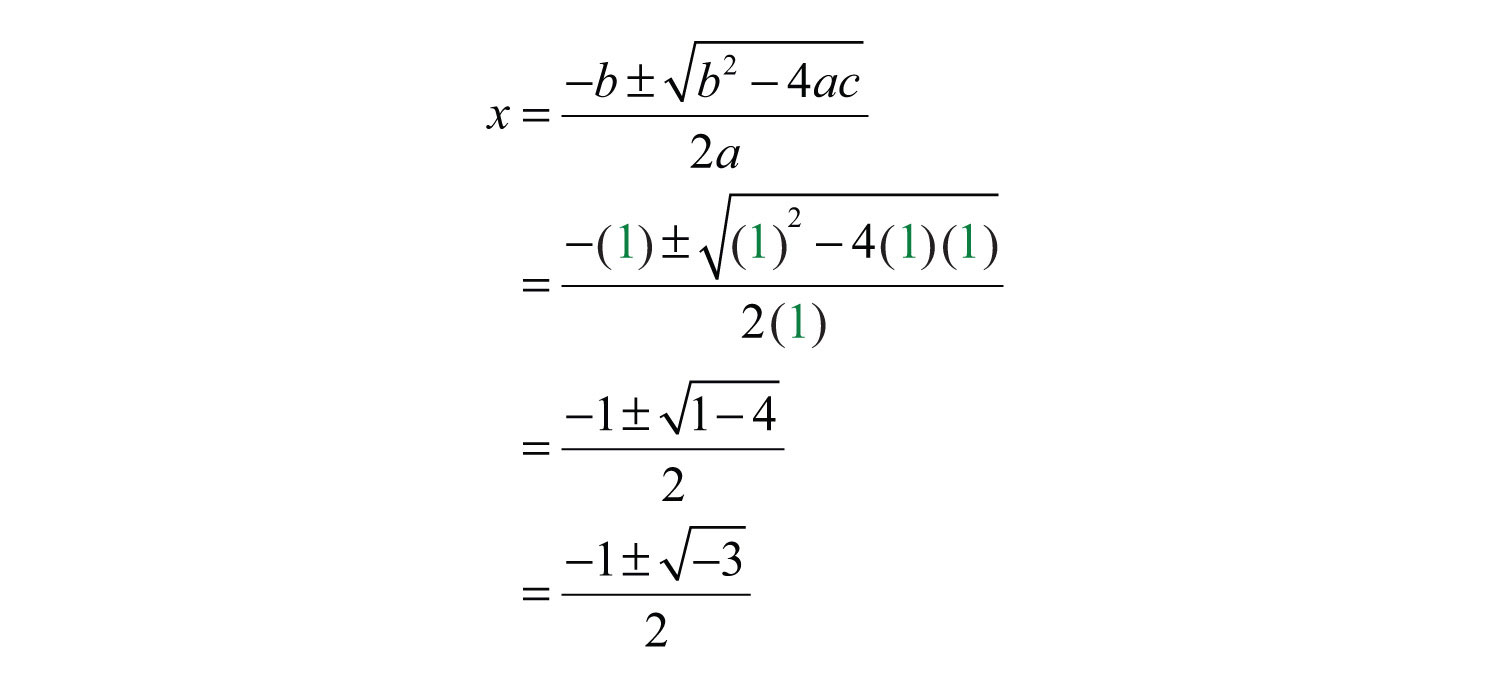The solution involves the square root of a negative number; hence the solutions are not real. This quadratic equation has two nonreal solutions and will be discussed in further detail as we continue in our study of algebra. For now, simply state that the equation does not have real solutions.

Try this! Solve: $x2−2x−2=0$.

Answer: $1±3$

### Video Solution

(click to see video)

It is important to place the quadratic equation in standard form before using the quadratic formula.

Example 6: Solve using the quadratic formula: $(2x+1)(2x−1)=24x+8$.

Solution: Begin by using the distributive property to expand the left side and combining like terms to obtain an equation in standard form, equal to 0.Once the equation is in standard form, identify a, b, and c. HereSubstitute these values into the quadratic formula and then simplify.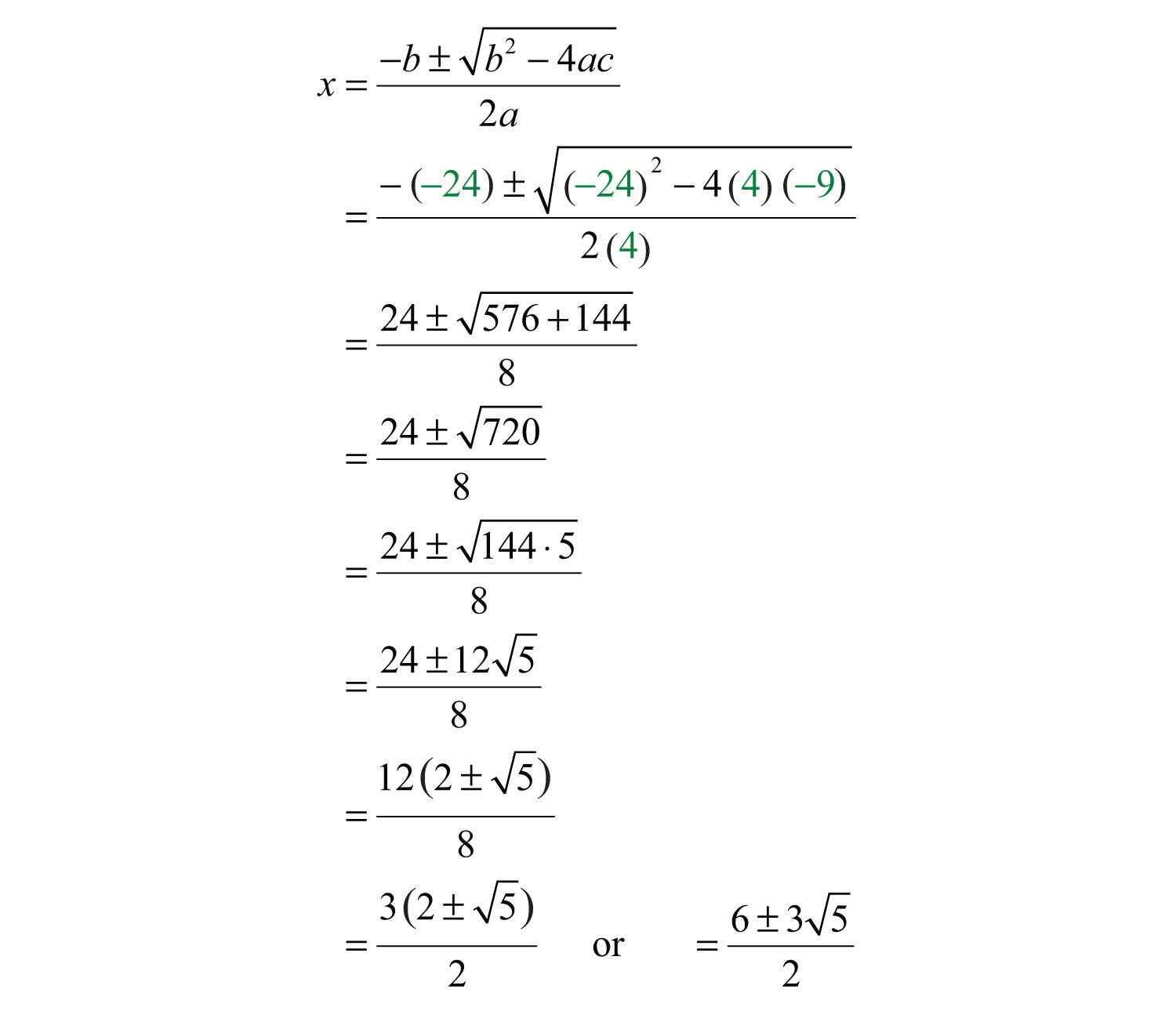Answer: The solutions are $6±352$.

Try this! Solve: $3x(x−2)=1$.

Answer: $3±233$

### Video Solution

(click to see video)

### Key Takeaways

• Use the quadratic formula to solve any quadratic equation in standard form.
• To solve any quadratic equation, first rewrite in standard form, $ax2+bx+c=0$, substitute the appropriate coefficients into the quadratic formula, $x=−b±b2−4ac2a$, and then simplify.

### Topic Exercises

Identify the coefficients a, b, and c used in the quadratic formula. Do not solve.

1. $x2−x+5=0$

2. $x2−3x−1=0$

3. $3x2−10=0$

4. $−y2+5=0$

5. $5t2−7t=0$

6. $−y2+y=0$

7. $−x2+x=−6$

8. $−2x2−x=−15$

9. $(3x+1)(2x+5)=19x+4$

10. $(4x+1)(2x+1)=16x+4$

Solve by factoring and then solve using the quadratic formula. Check answers.

11. $x2−10x+24=0$

12. $x2−3x−18=0$

13. $t2+6t+5=0$

14. $t2+9t+14=0$

15. $2x2−7x−4=0$

16. $3x2−x−2=0$

17. $−2x2−x+3=0$

18. $−6x2+x+1=0$

19. $y2−2y+1=0$

20. $y2−1=0$

Use the quadratic formula to solve the following.

21. $x2−6x+4=0$

22. $x2−4x+1=0$

23. $x2+2x−5=0$

24. $x2+4x−6=0$

25. $t2−4t−1=0$

26. $t2−8t−2=0$

27. $−y2+y+1=0$

28. $−y2−3y+2=0$

29. $−x2+16x−62=0$

30. $−x2+14x−46=0$

31. $2t2−4t−3=0$

32. $4t2−8t−1=0$

33. $−4y2+12y−9=0$

34. $−25x2+10x−1=0$

35. $3x2+6x+2=0$

36. $5x2+10x+2=0$

37. $9t2+6t−11=0$

38. $8t2+8t+1=0$

39. $x2−2=0$

40. $x2−18=0$

41. $9x2−3=0$

42. $2x2−5=0$

43. $y2+9=0$

44. $y2+1=0$

45. $2x2=0$

46. $x2=0$

47. $−2y2+5y=0$

48. $−3y2+7y=0$

49. $t2−t=0$

50. $t2+2t=0$

51. $x2−0.6x−0.27=0$

52. $x2−1.6x−0.8=0$

53. $y2−1.4y−0.15=0$

54. $y2−3.6y+2.03=0$

55. $12t2+5t+32=0$

56. $−t2+3t−34=0$

57. $3y2+12y−13=0$

58. $−2y2+13y+12=0$

59. $2x2−10x+3=4$

60. $3x2+6x+1=8$

61. $−2y2=3(y−1)$

62. $3y2=5(2y−1)$

63. $(t+1)2=2t+7$

64. $(2t−1)2=73−4t$

65. $(x+5)(x−1)=2x+1$

66. $(x+7)(x−2)=3(x+1)$

67. $x(x+5)=3(x−1)$

68. $x(x+4)=−7$

69. $(5x+3)(5x−3)−10(x−1)=0$

70. $(3x+4)(3x−1)−33x=−20$

71. $27y(y+1)+2(3y−2)=0$

72. $8(4y2+3)−3(28y−1)=0$

73. $(x+2)2−2(x+7)=4(x+1)$

74. $(x+3)2−10(x+5)=−2(x+1)$

Part B: Discussion Board

75. When talking about a quadratic equation in standard form, $ax2+bx+c=0$, why is it necessary to state that $a≠0$? What happens if a is equal to 0?

76. Research and discuss the history of the quadratic formula and solutions to quadratic equations.

1: $a=1$, $b=−1$, and $c=5$

3: $a=3$, $b=0$, and $c=−10$

5: $a=5$, $b=−7$, and $c=0$

7: $a=−1$, $b=1$, and $c=6$

9: $a=6$, $b=−2$, and $c=1$

11: 4, 6

13: −5, −1

15: −1/2, 4

17: −3/2, 1

19: 1, double root

21: $3±5$

23: $−1±6$

25: $2±5$

27: $1±52$

29: $8±2$

31: $2±102$

33: 3/2, double root

35: $−3±33$

37: $−1±233$

39: $±2$

41: $±33$

43: No real solutions

45: 0, double root

47: 0, 5/2

49: 0, 1

51: −0.3, 0.9

53: −0.1, 1.5

55: $−5±22$

57: $−1±1712$

59: $5±332$

61: $−3±334$

63: $±6$

65: $−1±7$

67: No real solutions

69: 1/5, double root

71: −4/3, 1/9

73: $1±15$